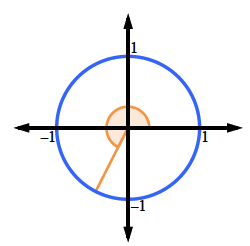### Home > CCA2 > Chapter Ch7 > Lesson 7.1.6 > Problem7-90

7-90.

Calculate the value of each expression below. Give an exact measurement, if possible. Each measure is given in radians.

1. $\sin(4)$

$−0.76$
1. $\sin(\frac{4\pi}{3})$
Since $\frac{4\pi}{3}$ is related to the $30^{^{\circ}}-60^{^{\circ}}-90^{^{\circ}}$ angles, this one has an exact answer.  Locate it on the unit circle. Notice that it will be a reflection (twice) of $\sin\left(\frac{\pi}{3}\right)$.
It is in the third quadrant.